## 2.13.2017

### Transformations of trigonometric functions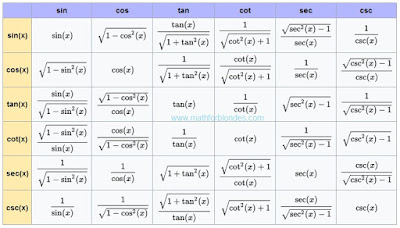Transformations of trigonometric functions
This table shows how one trigonometric functions can be transformed to other trigonometric functions. Sin, cos, tan, cot, sec, csc - all these functions can be transformed.

## 2.10.2017

### Number to the power minus one

What to do if number to the power minus one? Write down this number in a fraction denominator. Here several examples with numbers to the power minus one.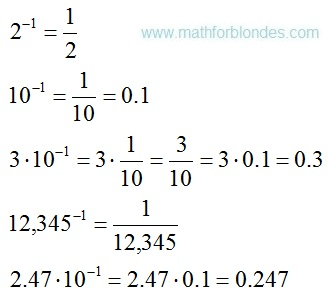Number to the power minus one
Negative exponents of numbers mean that these numbers are in a fraction denominator.Negative exponents

### Trigonometrical circle of a tangent

Last time I drew for you a unit circle of cotangent. Now you can look at a trigonometrical circle of tangent.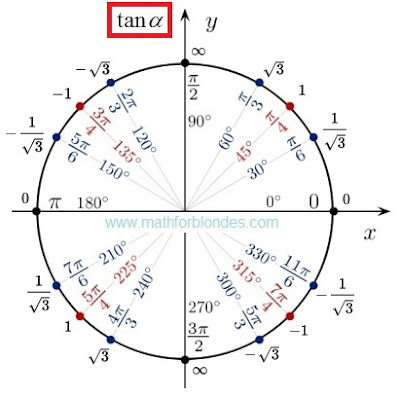Trigonometrical circle of a tangent
There is no sense here. There are angles and values of tangents. If you want to understand sense of a tangent and cotangent, look here.

## 2.08.2017

### Trigonometric tableTrigonometric table are crazy
This trigonometrical table is an example of mathematical marasmus. I am touched by exact values of a sine and cosine. No comments.

If mathematicians do not know what is trigonometric functions, let read here. If mathematicians are not able to divide into zero, let study. I like the idea of this table. I do not like its contents. I corrected this trigonometrical table.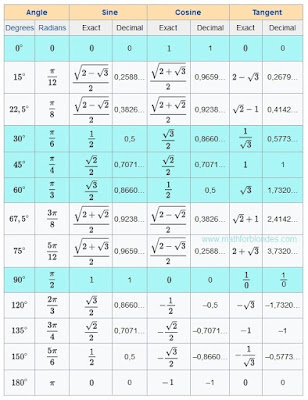Trigonometric table

The most popular angles are highlighted with blue color. 0, 30, 45, 60, 90 degrees most often occur in textbooks.Common fractions will be useful to pupils to fight against teachers. Decimal fractions will be useful physics and to engineers to calculations.

In this trigonometrical table there are no cotangents (cot, cotan, cotg, ctg, ctn). Anything terrible. There are useful formulas which will help you.Useful formulas

Sin 0, 15, 22.5, 30, 45, 60, 67.5, 75, 90, 120, 135, 150, 180 degrees in this table.
Cos 0, Pi/12, pi/8, pi/6, pi/4, pi/3, 3/8 pi, 5/12 pi, pi/2, 2/3 pi, 3/4 pi, 5/6 pi, 1 pi radians.
Tan pi/2 radians or 90 degrees it makes sense.

### Cotangent 210 degrees

Today we will consider a cotangent of 210 degrees. We will draw a unit disk and we will write values of cotangents. Here what turned out.The unit circle and cotangent
Value of a cotangent of 210 degrees same, as at a cotangent of 30 degrees. We take mathematics in hand and we check. The cotangent is the cosine divided into a sine. Values of a sine and cosine of 30 and 210 degrees can be taken here.Cotangent 210 degrees
We already know how to divide fraction into fraction. Formulas of transformation of angles of trigonometric functions.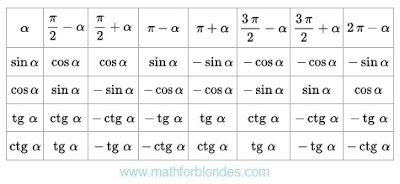Formulas of transformation of angles
These formulas will be very useful to you.

## 2.07.2017

### Signs of trigonometric functions

All trigonometric functions can have different signs. Signs of trigonometric functions depend on the coordinate system. In mathematician the Cartesian coordinate system is accepted. Four quadrants of a Cartesian coordinate system define signs of trigonometric functions.Signs of trigonometric functions
You do not speak to mathematicians, but remember. Signs are not property of trigonometric functions. Without coordinate system signs will not be. If to measure corners on another, signs will be others. In other coordinate system signs can be others.Signs of trigonometric functions are a property of the chosen coordinate system. Functions are considered in mathematician only in the Cartesian coordinate system.

## 2.06.2017

### How to divide fraction into fraction?

When performing calculations often there is a question: "How to divide fraction into fraction?". Mathematicians rules tell so:

To divide a number by a fraction, multiply that number by the reciprocal of that fraction.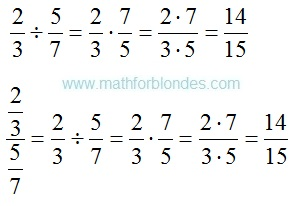How to divide fraction into fraction?
Transform fraction to division if in numerator and a denominator there are fractions. Be attentive! The numerator and denominator of fraction need to be known. The result depends on it.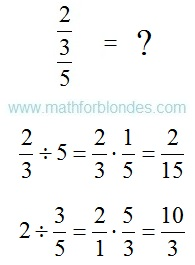The numerator and denominator

As fraction to divide into fraction. Formula of division of fractions.Formula of division of fractions
You believe that in mathematician there is a division? The fraction cannot be divided into fraction. They can only be multipled.